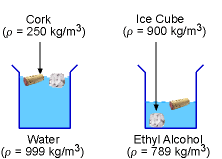Ch 1. Basics Multimedia Engineering Fluids MassDensity IdealGas Law Viscosity SurfaceTension VaporPressure
 Chapter 1. Basics 2. Fluid Statics 3. Kinematics 4. Laws (Integral) 5. Laws (Diff.) 6. Modeling/Similitude 7. Inviscid 8. Viscous 9. External Flow 10. Open-Channel Appendix Basic Math Units Basic Equations Water/Air Tables Sections Search eBooks Dynamics Fluids Math Mechanics Statics Thermodynamics Author(s): Chean Chin Ngo Kurt Gramoll ©Kurt GramollFLUID MECHANICS - CASE STUDY SOLUTION

The raw data collected are given as follows:

 Empty Container Mass (g) Container & Liquid Mass (g) Volume of Liquid (ml) Liquid A 25.0 75.0 50 Liquid B 25.0 44.7 25

The mass of the liquid is determined by subtracting the mass of the empty container from the mass of the container with liquid. The density of the liquid is then determined by dividing the mass by its volume.

For Liquid A:
Mass of the Liquid = 75 - 25 = 50 g
Density = ρA = m/V
= 50 g / 50 ml = 1 g/ml
= 1000 kg/m3

For Liquid B:
Mass of the Liquid = 44.7 - 25 = 19.7 g
Density = ρB = m/V
= 19.7 g / 25 ml = 0.788 g/ml
= 788 kg/m3Ice Cube and Cork in
Water and Ethyl Alcohol

By comparing the computed densities with the density values found in standard handbooks, it is possible that liquid A is water while liquid B is ethyl alcohol (note that other liquids or mixtures may have the same densities).

The density of the ice cube (900 kg/m3) is less than the water's density, but it is greater than the ethyl alcohol's density. Hence, the ice cube will float on water while it will sink in ethyl alcohol.

On the other hand, a cork of density 250 kg/m3 is less dense than the water and the ethyl alcohol. Hence, it will float on both the liquids.

Practice Homework and Test problems now available in the 'Eng Fluids' mobile app
Includes over 250 problems with complete detailed solutions.
Available now at the Google Play Store and Apple App Store.